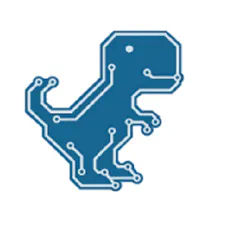# DIY Simple Autorange Capacitance Meter (10pF-10000microF)

Inexpensive, precision, autorange capacitance meter with a large range.

BeginnerFull instructions provided26,644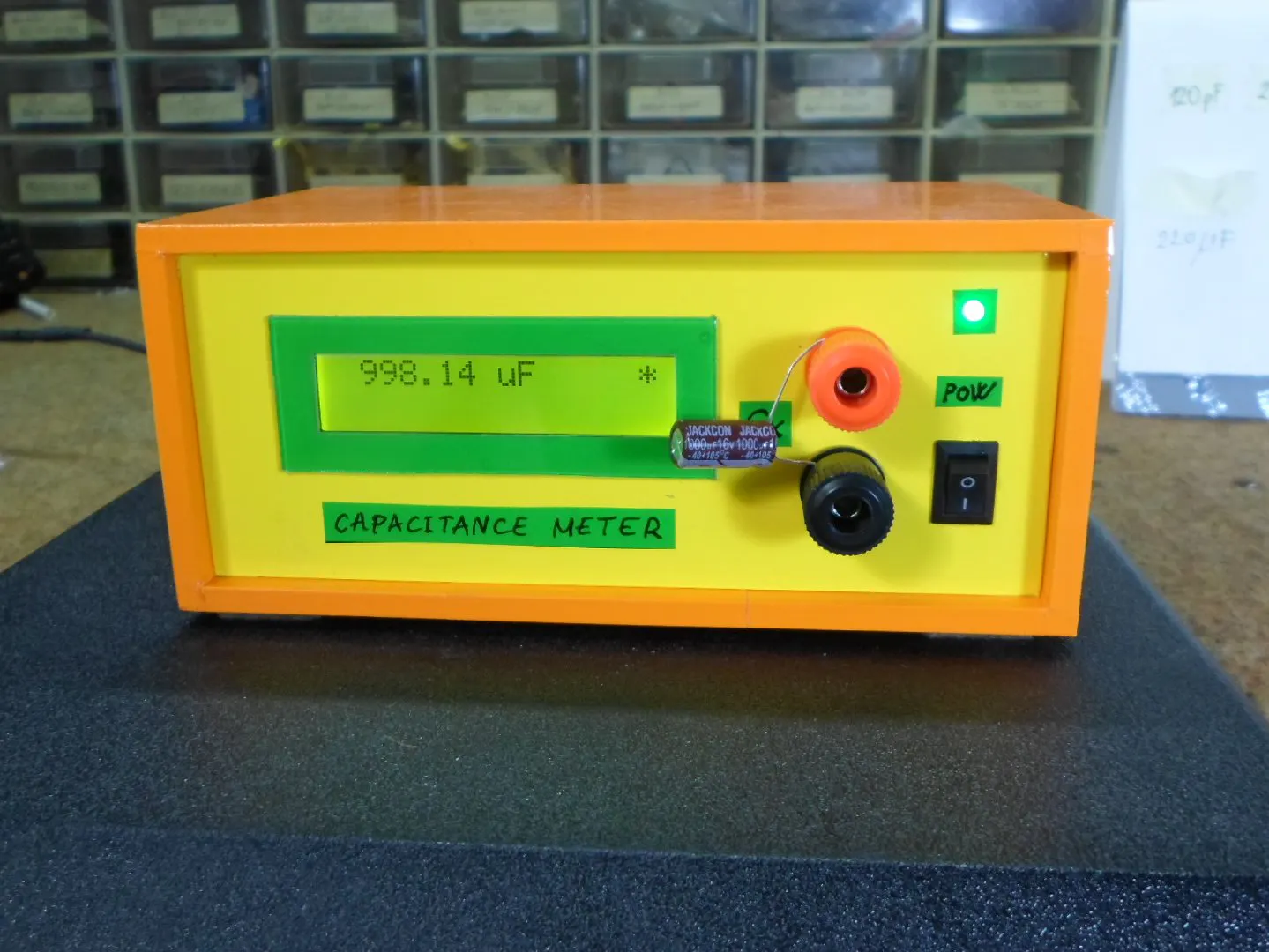## Things used in this project

### Hardware componentsArduino Nano R3
×1Resistor 10k ohm
×1Resistor 1k ohm
×1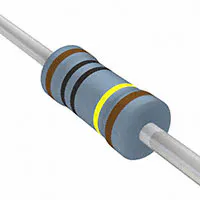Resistor 1M ohm
×1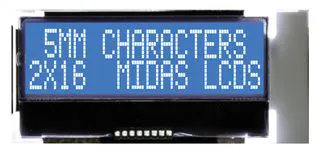Alphanumeric LCD, 16 x 2
×1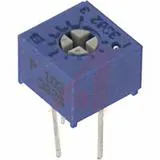Single Turn Potentiometer- 10k ohms
×1

### Software apps and online servicesArduino IDE

### Hand tools and fabrication machinesSoldering iron (generic)

## Schematics

### Schematic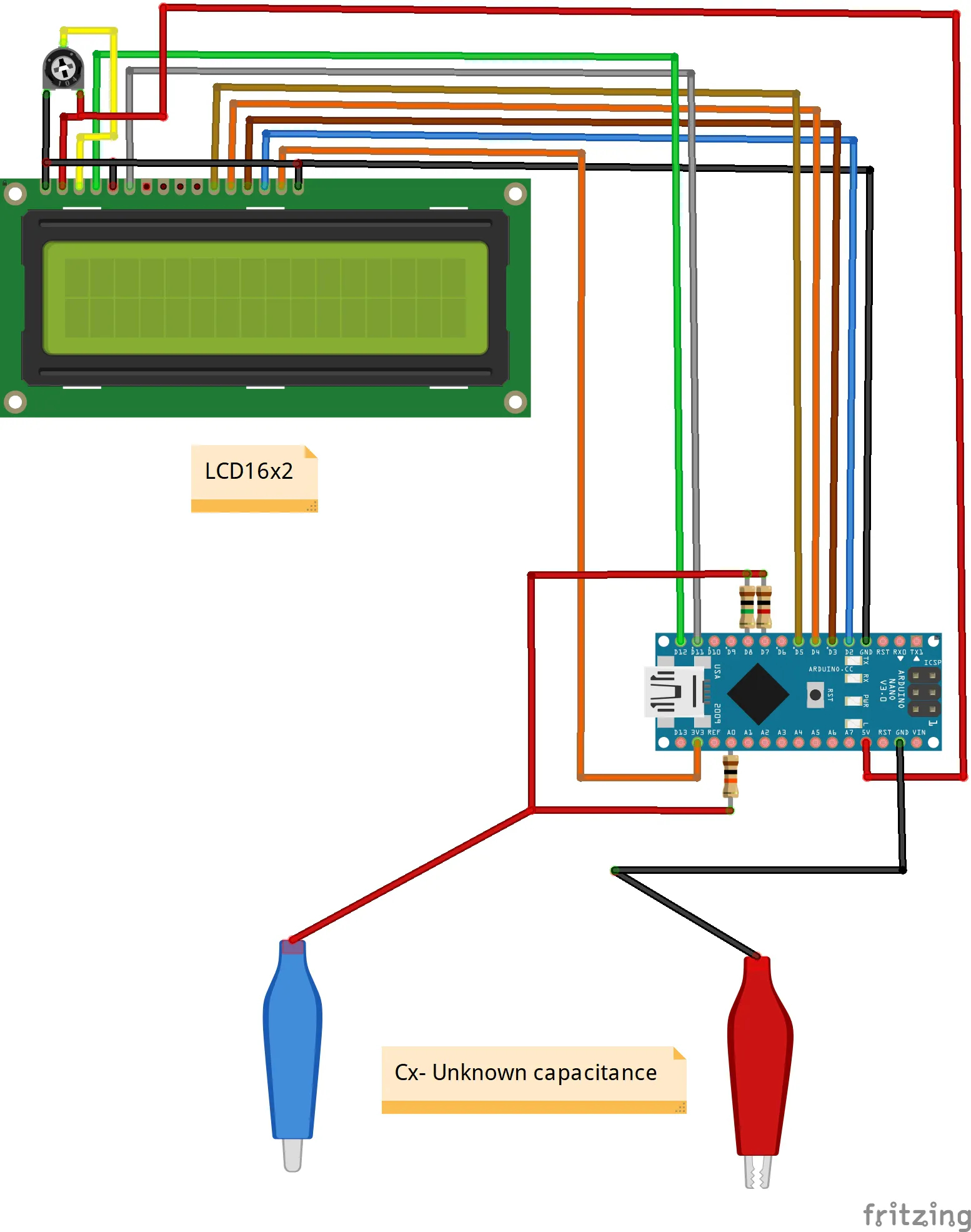## Code

### Arduino code

C/C++
```#include <LiquidCrystal.h>
LiquidCrystal lcd(12, 11, 5, 4, 3, 2);// RS,E,D4,D5,D6,D7
void setup(){
lcd.begin(16, 2);// LCD 16X2
pinMode(A0,INPUT);
}
unsigned long time0,time1,time2;
float c,null0;
byte kn,mk,i;

void loop(){
lcd.setCursor(15,0);
lcd.print("*");
if(mk==0){
pinMode(8,OUTPUT);
pinMode(7,INPUT);
digitalWrite(8,HIGH);
}

if(mk==1){
pinMode(7,OUTPUT);
pinMode(8,INPUT);
digitalWrite(7,HIGH);
}

time0=micros();
time2=micros()-time0;
if(time2>=1000000 && mk==0){
mk=1;
time0=100000000;
break;
}
}

time1=micros()-time0;

pinMode(7,OUTPUT);
pinMode(8,OUTPUT);
digitalWrite(7,LOW);
digitalWrite(8,LOW);
}

if(mk==1&&time1<1000){
mk=0;
}

lcd.setCursor(1,0);
c=time1;
c=c/1000-null0;
c=abs(c);

if(time1>=10000000){
lcd.setCursor(1,0);
lcd.print(" TEST uF   ");
}
else{
lcd.print(c);

if(mk==0){
lcd.print(" nF       ");
}
if(mk==1){
lcd.print(" uF       ");
}
}
if(i==0){
i++;
null0=c+0.02;
}
delay(100);
}
```

## Credits

### Mirko Pavleski

76 projects • 997 followers